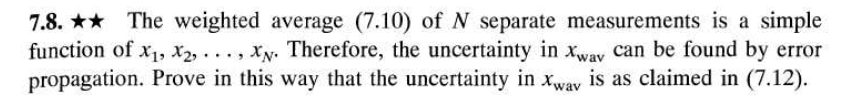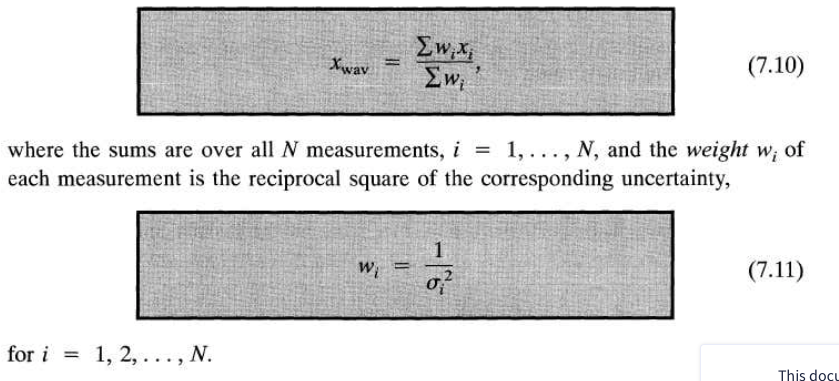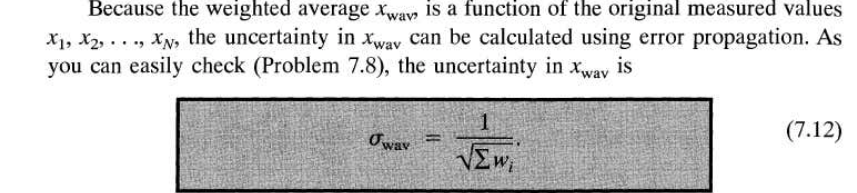# Question (7.10): (7.12): 7.8. ** The weighted average (7.10) of N separate measurements is a simple function of x1, x2, ..., xy. Therefore, the uncertainty in Xwav can be found by error propagation. Prove in this way that the uncertainty in xway is as claimed in (7.12). Ew;x; Ew;? (7.10) where the sums are over all N measurements, i = 1, ...,N, and the weight w; of each measurement is the reciprocal square of the corresponding uncertainty, (7.11) for i = 1, 2, ..., N. This docu Because the weighted average Xway, is a function of the original measured values X1, X2, ..., Xy, the uncertainty in Xway can be calculated using error propagation. As you can easily check (Problem 7.8), the uncertainty in Xway is (7.12) Oway =D9MT5I The Asker · Probability and Statistics(7.10):(7.12):Transcribed Image Text: 7.8. ** The weighted average (7.10) of N separate measurements is a simple function of x1, x2, ..., xy. Therefore, the uncertainty in Xwav can be found by error propagation. Prove in this way that the uncertainty in xway is as claimed in (7.12). Ew;x; Ew;? (7.10) where the sums are over all N measurements, i = 1, ...,N, and the weight w; of each measurement is the reciprocal square of the corresponding uncertainty, (7.11) for i = 1, 2, ..., N. This docu Because the weighted average Xway, is a function of the original measured values X1, X2, ..., Xy, the uncertainty in Xway can be calculated using error propagation. As you can easily check (Problem 7.8), the uncertainty in Xway is (7.12) Oway =
More
Transcribed Image Text: 7.8. ** The weighted average (7.10) of N separate measurements is a simple function of x1, x2, ..., xy. Therefore, the uncertainty in Xwav can be found by error propagation. Prove in this way that the uncertainty in xway is as claimed in (7.12). Ew;x; Ew;? (7.10) where the sums are over all N measurements, i = 1, ...,N, and the weight w; of each measurement is the reciprocal square of the corresponding uncertainty, (7.11) for i = 1, 2, ..., N. This docu Because the weighted average Xway, is a function of the original measured values X1, X2, ..., Xy, the uncertainty in Xway can be calculated using error propagation. As you can easily check (Problem 7.8), the uncertainty in Xway is (7.12) Oway =